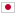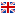Contest Duration: - (local time) (300 minutes) Back to Home
I - ADD DIV MAX RESTORE/Time Limit: 3 sec / Memory Limit: 1024 MB

Score : 100 points

### Problem Statement

You are given an integer sequence a_0, a_1, ..., a_{N-1}.

You have to perform Q queries, each query is one of the following:

• ADD QUERY(t=0 l r x) : for each i between l and r, inclusive, replace a_i with a_i + x.
• DIV QUERY(t=1 l r x) : for each i between l and r, inclusive, replace a_i with {\rm floor}(a_i / x), where {\rm floor}(y) is the biggest integer that is not greater than y.
• MAX QUERY(t=2 l r x=0) : print {\rm max}(a_l, a_{l+1}, ..., a_r).
• RESTORE QUERY(t=3 l r x=0) : for each i between l and r, inclusive, set a_i to the initial value of a_i, that is, the value given in the input.

### Constraints

• All input values are integers.
• 1 \leq N, Q \leq 200,000
• 0 \leq a_i \leq 10^8
• t_i = 0, 1, 2, 3
• 0 \leq l_i \leq r_i \leq N-1
• 1 \leq x_i \leq 1000(when t_i \neq 2, 3)

### Input

Input is given from Standard Input in the following format:

N Q
a_0 a_1 ... a_{N-1}
t_1 l_1 r_1 x_1
t_2 l_2 r_2 x_2
:
t_Q l_Q r_Q x_Q


### Output

For each MAX QUERY, print {\rm max}(a_l, a_{l+1}, ..., a_r), one per line.

### Sample Input 1

5 9
1 2 3 4 5
2 0 4 0
0 0 1 10
2 0 4 0
2 2 2 0
1 0 1 4
2 0 0 0
2 1 1 0
3 0 4 0
2 0 1 0


### Sample Output 1

5
12
3
2
3
2

• {\rm max}(1, 2, 3, 4, 5) = 5
• 1, 2, 3, 4, 5 → 11, 12, 3, 4, 5
• {\rm max}(11, 12, 3, 4, 5) = 12
• {\rm max}(3) = 3
• 11, 12, 3, 4, 5 → 2, 3, 3, 4, 5
• {\rm max}(2) = 2
• {\rm max}(3) = 3
• The array is restored to 1, 2, 3, 4, 5
• {\rm max}(1, 2) = 2

### Sample Input 2

4 7
0 1 0 1
2 0 3 0
0 0 3 1
1 0 3 2
2 0 3 0
0 0 3 1
1 0 3 2
2 0 3 0


### Sample Output 2

1
1
1


### Sample Input 3

10 23
13 1 22 8 28 18 23 9 22 27
1 3 4 5
1 8 8 8
0 3 9 5
0 2 6 3
3 0 4 0
1 1 3 7
2 2 2 0
2 3 5 0
0 1 4 2
3 0 9 0
2 0 1 0
0 3 9 8
2 1 9 0
0 8 9 5
1 5 7 7
0 3 5 7
0 7 9 7
3 3 6 0
2 1 6 0
0 1 1 7
1 4 8 10
2 0 9 0
1 5 6 1


### Sample Output 3

3
28
13
36
28
47